#DAY 21
3
Software Development

## Day21- 黑魔法，recursion，recursion depon (遞迴函數的介紹)(圖片來源: 截自動畫「魔法咪路咪路」)

# 遞迴函數工作原理?

## 範例21-1: 階乘

`n! = 1*2*3*…*(n-1)*n`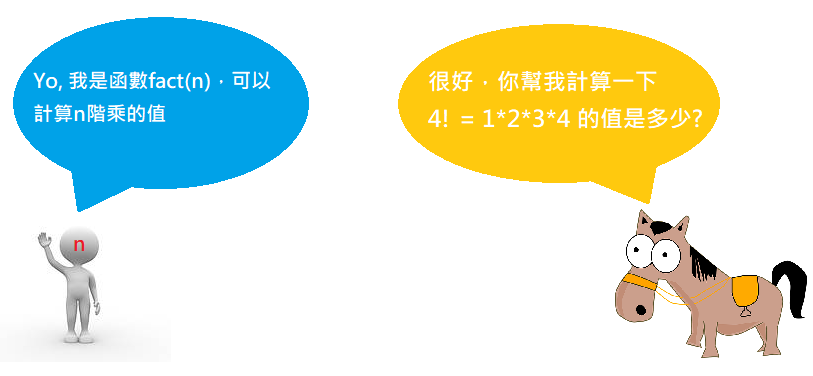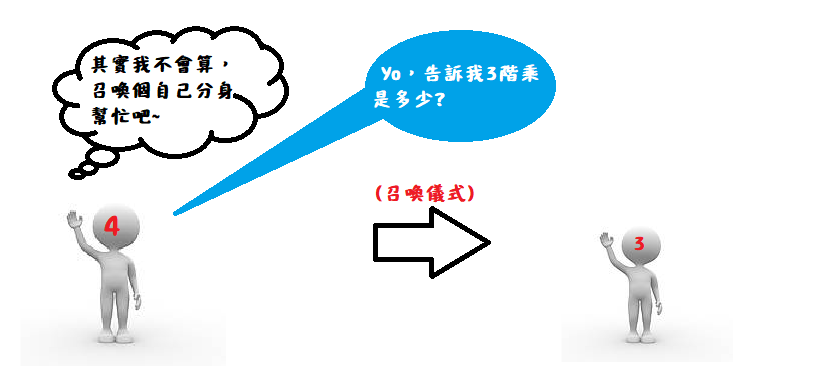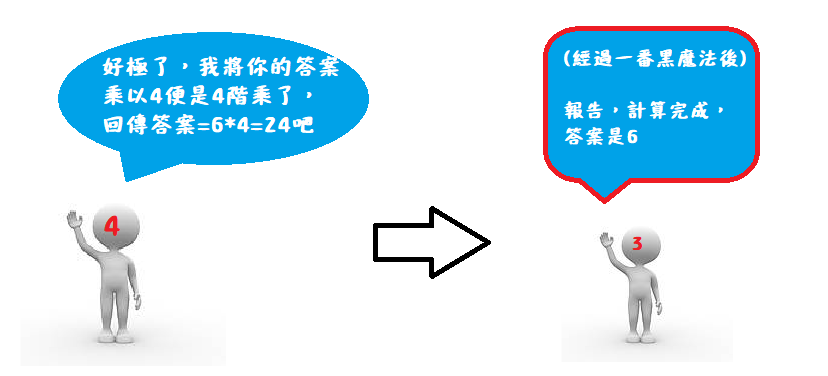`fact(4)`只要把它拿到的答案再乘上`4`就是答案了。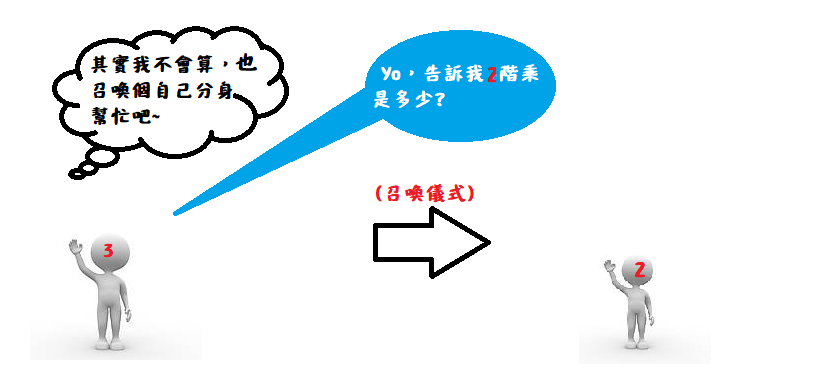`fact(2)`會告訴`fact(3)`說2階乘答案是2，
`fact(3)`他只要把2乘上3就得到6這個答案了。

(階乘的英文是factorial，這邊函數名取簡寫叫fact)

``````def fact(n):
return fact(n-1)*n

print(fact(4))
``````

## 遞迴函數兩大要素: 「遞迴關係」與「終止條件」

1. 遞迴關係，也就是本文所稱的「黑魔法」，指函數之間大問題與小問題之間的關係，如上例中的`fact(n)=fact(n-1)*n`即是一例。
2. 終止條件，指遞迴關係的停止條件，例如上例的條件應為`fact(1)=1`

`fact(0)`又會去問`fact(-1)`-1階乘是多少，

``````def fact(n):
if n==1:
return 1
return n*fact(n-1)
``````

``````def fact(n):
return 1 if n==1 else n*fact(n-1)
``````

## 範例21-2: 河內塔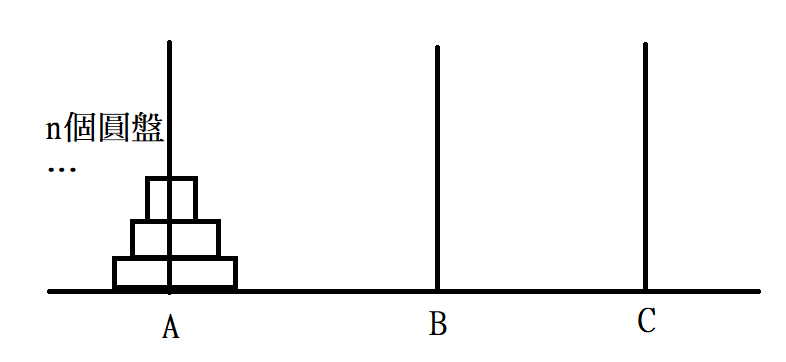1. 將A石柱的前n-1個金片搬到B石柱上
2. 將A石柱最大的金片搬到C石柱上
3. 將B石柱的n-1個金片搬到C石柱上

``````def hanoi(n,a,b,c): #將石柱a的n個圓盤移到石柱c(b為輔助)
if n==1:
print(f"將1號金片由{a}石柱移動到{c}石柱")
else:
hanoi(n-1,a,c,b)
print(f"將{n}號金片由{a}石柱移動到{c}石柱")
hanoi(n-1,b,a,c)

hanoi(4,'A','B','C')
``````

``````將1號金片由A石柱移動到B石柱

``````

## 範例21-3: 爬樓梯問題

1. 每次都爬一階
2. 先爬一階再爬兩階
3. 先爬兩階再爬一階

(註: 為什麼爬0階階梯算只有一種方法呢?

``````def stair(n): #注意: 執行速度非常慢
return stair(n-1)+ stair(n-2) if n>=2 else 1
``````

``````def stair_fast(n):
ways = * (n+1) #用一個列表記錄爬到第i階階梯共幾種方法
ways = 1 # 初始條件
ways = 1 # 初始條件
for i in range(2,n+1):
ways[i]=ways[i-1]+ways[i-2]
return ways[-1]
``````1